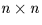Next: SLA_SUBET - Remove E-terms
Up: SUBPROGRAM SPECIFICATIONS
Previous: SLA_SEP - Angle Between 2 Points on Sphere

## SLA_SMAT - Solve Simultaneous Equations

ACTION:
Matrix inversion and solution of simultaneous equations (single precision).

CALL:
CALL sla_SMAT (N, A, Y, D, JF, IW)

GIVEN:

 I N number of unknowns A R(N,N) matrix Y R(N) vector

RETURNED:

 R(N,N) A matrix inverse Y R(N) solution D R determinant JF I singularity flag: 0=OK IW I(N) workspace

NOTES:
1.
For the set of n simultaneous linear equations in n unknowns:
Ay = x
where:
• A is a non-singularmatrix,
• y is the vector of n unknowns, and
• x is the known vector,
sla_SMAT computes:
• the inverse of matrix A,
• the determinant of matrix A, and
• the vector of n unknowns y.
Argument N is the order n, A (given) is the matrix A, Y (given) is the vector x and Y (returned) is the vector y. The argument A (returned) is the inverse matrix A-1, and D is det(A).
2.
JF is the singularity flag. If the matrix is non-singular, JF=0 is returned. If the matrix is singular, JF=-1 and D=0.0 are returned. In the latter case, the contents of array A on return are undefined.
3.
The algorithm is Gaussian elimination with partial pivoting. This method is very fast; some much slower algorithms can give better accuracy, but only by a small factor.
4.
This routine replaces the obsolete sla_SMATRX.Next: SLA_SUBET - Remove E-terms
Up: SUBPROGRAM SPECIFICATIONS
Previous: SLA_SEP - Angle Between 2 Points on Sphere

SLALIB --- Positional Astronomy Library